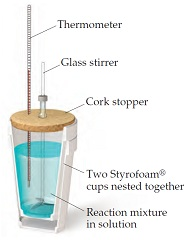# Problem: When a 6.50-g sample of solid sodium hydroxide dissolves in 100.0 g of water in a coffee-cup calorimeter (the following Figure), the temperature rises from 21.6 oC to 37.8 oC.Calculate ΔH (in kJ/mol NaOH) for the solution processNaOH(s) → Na+(aq) + OH-(aq)Assume that the specific heat of the solution is the same as that of pure water.

###### FREE Expert Solution

We are asked to calculate ΔH (in kJ/mol NaOH) for the solution process.

Calculate heat:

Q = 6.78 kJ

100% (180 ratings)###### Problem Details
When a 6.50-g sample of solid sodium hydroxide dissolves in 100.0 g of water in a coffee-cup calorimeter (the following Figure), the temperature rises from 21.6 oC to 37.8 oC.Calculate ΔH (in kJ/mol NaOH) for the solution process

NaOH(s) → Na+(aq) + OH-(aq)

Assume that the specific heat of the solution is the same as that of pure water.

Frequently Asked Questions

What scientific concept do you need to know in order to solve this problem?

Our tutors have indicated that to solve this problem you will need to apply the Constant-Pressure Calorimetry concept. You can view video lessons to learn Constant-Pressure Calorimetry. Or if you need more Constant-Pressure Calorimetry practice, you can also practice Constant-Pressure Calorimetry practice problems.

What professor is this problem relevant for?

Based on our data, we think this problem is relevant for Professor Liwosz's class at UB.